# 一、分类算法评价指标

## 2.混淆矩阵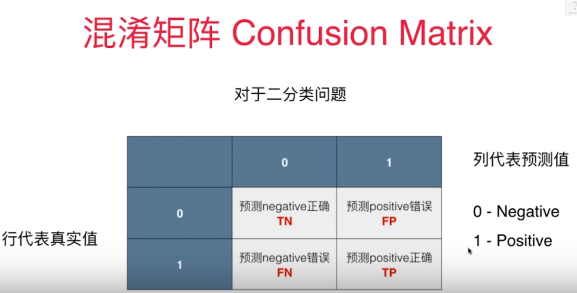## 3.精准率和召回率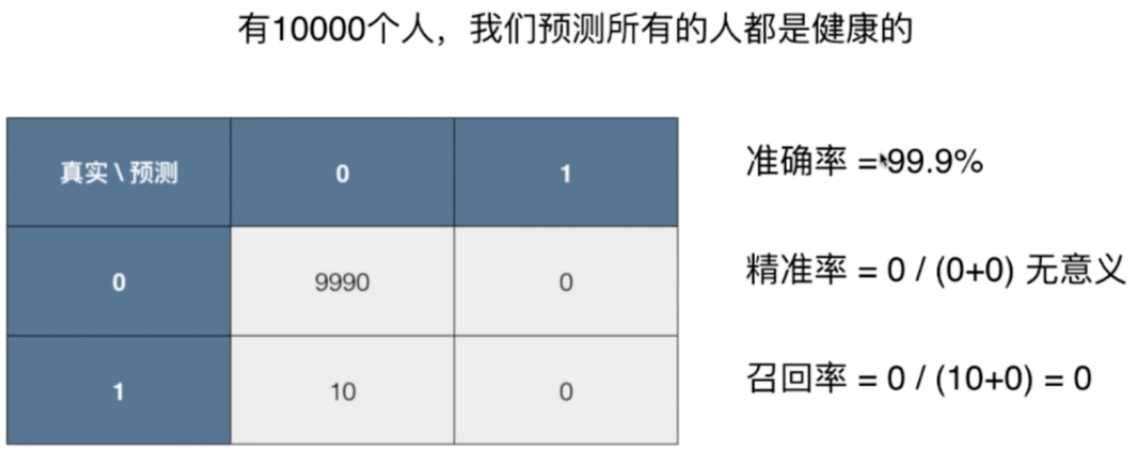## 4.分类算法常见的评估指标如下

• 对于二类分类器/分类算法，评价指标主要有accuracy， [Precision，Recall，F-score，Pr曲线]，ROC-AUC曲线。
• 对于多类分类器/分类算法，评价指标主要有accuracy， [宏平均和微平均，F-score]。

## 5.分类指标评价计算示例

### 1)精准率和召回率

#### Precision

# precision
import numpy as np
from sklearn.metrics import precision_score
y_true = [1,2,3,4]
y_pre = [1,2,4,3]
print('precision指标为:',precision_score(y_true,y_pre,average='micro'))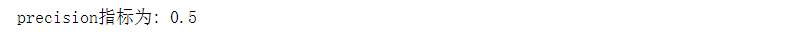#### Recall

#recall
import numpy as np
from sklearn.metrics import recall_score
y_true = [1,2,3,4]
y_pre = [1,2,4,3]
print('recall指标为:',recall_score(y_true,y_pre,average='micro'))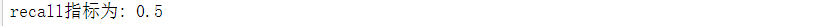### 2）F1-score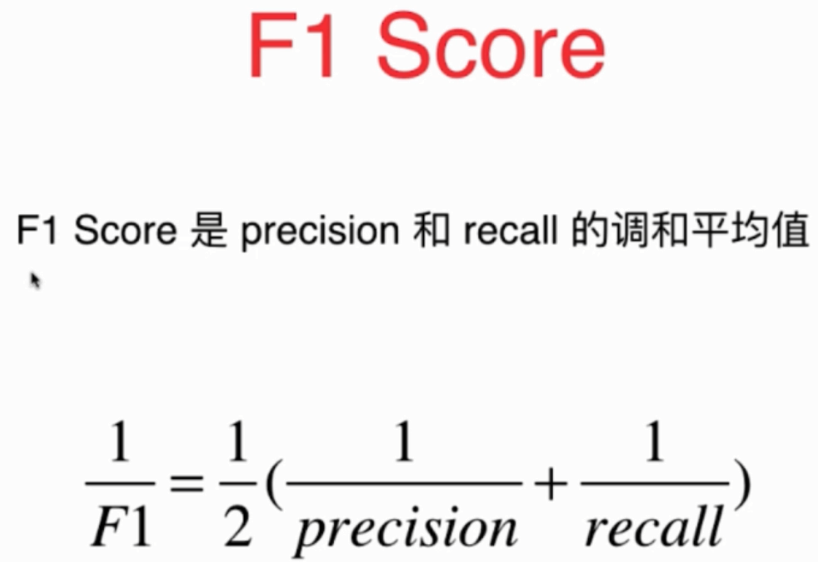#### F1-score

#F1-score
import numpy as np
from sklearn.metrics import f1_score
y_true = [1,2,3,4]
y_pre = [1,2,4,3]
print('f1指标为:',f1_score(y_true,y_pre,average='micro'))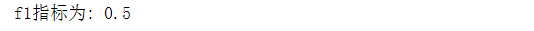### 3)ROC曲线的AUC

ROC曲线是用来描述TPR与FPR之间的曲线之间的关系.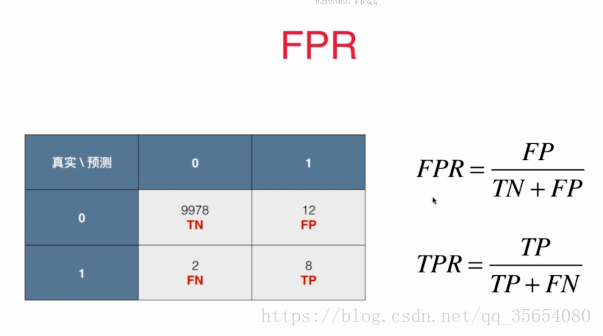TPR（True-Positive-Rate）: 代表预测为1并且预测对了的样本数量占真实值为1的百分比为多少

FPR（False-Positive-Rate）：代表预测为1但预测错了的样本数量占真实值为0的百分比是多少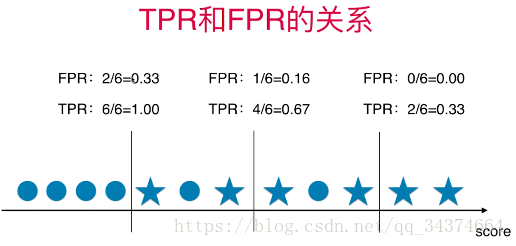#### 单纯的计算AUC

## AUC
import numpy as np
from sklearn.metrics import roc_auc_score
y_true = np.array([0, 0, 1, 1])
y_scores = np.array([0.1, 0.4, 0.35, 0.8])
print('AUC socre:',roc_auc_score(y_true, y_scores))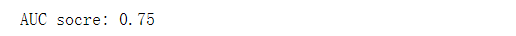#### 绘制ROC曲线

##### 封装TPR和FPR
def TPR(y_true, y_predict):
tp = TP(y_true, y_predict)
fn = FN(y_true, y_predict)
try:
return tp / (tp + fn)
except:
return 0.

def FPR(y_true, y_predict):
fp = FP(y_true, y_predict)
tn = TN(y_true, y_predict)
try:
return fp / (fp + tn)
except:
return 0.

##### 求TPR和FPR
import numpy as np
from sklearn import datasets

X = digits.data
y = digits.target.copy()
y[digits.target==9] = 1
y[digits.target!=9] = 0

from sklearn.model_selection import train_test_split
X_train, X_test, y_train, y_test = train_test_split(X, y, random_state=666)

from sklearn.linear_model import LogisticRegression
log_reg = LogisticRegression()
log_reg.fit(X_train, y_train)
decision_scores = log_reg.decision_function(X_test)

from playML.metrics import FPR, TPR

fprs = []
tprs = []
thresholds = np.arange(np.min(decision_scores), np.max(decision_scores), 0.1)

for threshold in thresholds:
# dtype=‘int‘：将数据类型从 bool 型转为 int 型；
y_predict = np.array(decision_scores >= threshold, dtype=‘int‘)
fprs.append(FPR(y_test, y_predict))
tprs.append(TPR(y_test, y_predict))

##### 绘制 ROC 曲线：
import matplotlib.pyplot as plt
plt.plot(fprs, tprs)
plt.show()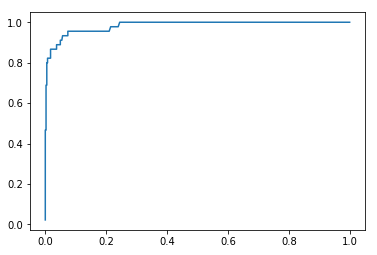ROC曲线的面积越大，那么我们的模型就越好，因为如果绘制一个FPR-TPR的曲线，在同样的FPR（犯错率）之下，TPR的值越高，模型越好，相应的它的面积越大。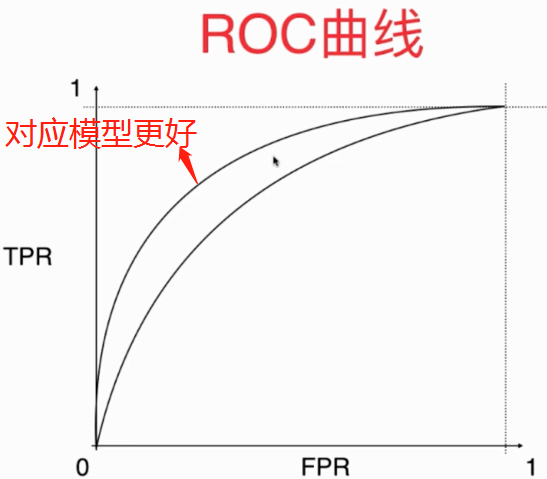from sklearn.metrics import roc_auc_score #求面积， 面积最大就为1，因为TPR与FPR最大值都为1
roc_auc_score(y_test, decision_scores)# 结果：0.98304526748971188


ROC对有偏数据并不敏感，主要用来比较两个模型谁更好一些，比如一个算法不同参数，或者两个不同的算法，都可以用这个来比较，如果他们的数据不是极度偏移的话。

# 二、多分类评价

## 1.多分类问题中的混淆矩阵

import numpy as np
import matplotlib.pyplot as plt

from sklearn import datasets
X = digits.data #数据并没有进行切割，所以这是10分类问题
y = digits.target

from sklearn.model_selection import train_test_split
X_train, X_test, y_train, y_test = train_test_split(X, y, test_size=0.8, random_state=666)

from sklearn.linear_model import LogisticRegression
log_reg = LogisticRegression()
log_reg.fit(X_train, y_train)
log_reg.score(X_test, y_test)

y_predict = log_reg.predict(X_test)

from sklearn.metrics import precision_score
precision_score(y_test, y_predict) #如果直接获取矩阵，会报错


ValueError: Target is multiclass but average=‘binary’. Please choose another average setting.



precision_score(y_test, y_predict, average="micro") #根据错误提示，需要更改参数
#为了求出多分类的精准度和召回率，必须传入参数average='micro'
from sklearn.metrics import confusion_matrix
confusion_matrix(y_test, y_predict) #跟2分类的混淆矩阵的含义一样，行代表真实值列代表预测值


View Code

cfm = confusion_matrix(y_test, y_predict)
plt.matshow(cfm, cmap=plt.cm.gray) #一个矩阵的绘制参数，第一个参数是矩阵，第二个参数是颜色
plt.show()


from sklearn.metrics import confusion_matrix
cfm = confusion_matrix(y_test,y_predict)
row_sums = np.sum(cfm,axis=1)#将每行相加，得到每行的样本总数
err_matrix = cfm/row_sums#绘制比例
np.fill_diagonal(err_matrix,0)# 因为对角线肯定对的多，将对角线的元素全部置为0
plt.matshow(err_matrix,cmap=plt.cm.gray) #越亮代表值越大，也就是错分的越多
plt.show()#这样就看出把什么看成什么的错误多了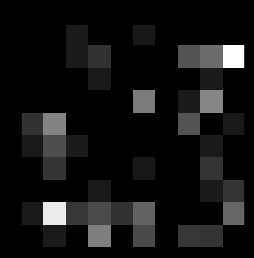©️2019 CSDN 皮肤主题: 酷酷鲨 设计师: CSDN官方博客